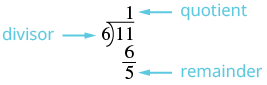## Converting Between Improper Fractions and Mixed Numbers

### Learning Outcomes

• Convert mixed numbers to improper fractions
• Convert improper fractions to mixed numbers

In an earlier example, we converted the improper fraction ${\Large\frac{11}{6}}$ to the mixed number $1{\Large\frac{5}{6}}$ using fraction circles. We did this by grouping six sixths together to make a whole; then we looked to see how many of the $11$ pieces were left. We saw that ${\Large\frac{11}{6}}$ made one whole group of six sixths plus five more sixths, showing that ${\Large\frac{11}{6}}=1{\Large\frac{5}{6}}$.

The division expression ${\Large\frac{11}{6}}$ (which can also be written as $6\overline{)11}$ ) tells us to find how many groups of $6$ are in $11$. To convert an improper fraction to a mixed number without fraction circles, we divide.

### Example

Convert ${\Large\frac{11}{6}}$ to a mixed number.

Solution:

 ${\Large\frac{11}{6}}$ Divide the denominator into the numerator. Remember ${\Large\frac{11}{6}}$ means $11\div 6$Identify the quotient, remainder and divisor. Write the mixed number as $\text{quotient }({\Large\frac{\text{remainder}}{\text{divisor}}})$ . $1{\Large\frac{5}{6}}$ So, ${\Large\frac{11}{6}}=1{\Large\frac{5}{6}}$

### Convert an improper fraction to a mixed number.

1. Divide the denominator into the numerator.
2. Identify the quotient, remainder, and divisor.
3. Write the mixed number as quotient ${\Large\frac{\text{remainder}}{\text{divisor}}}$ .

### Example

Convert the improper fraction ${\Large\frac{33}{8}}$ to a mixed number.

### try it

Now you can watch worked examples of how to convert an improper fraction to a mixed number in the following video.

In an earlier example, we changed $1{\Large\frac{4}{5}}$ to an improper fraction by first seeing that the whole is a set of five fifths. So we had five fifths and four more fifths.

${\Large\frac{5}{5}}+{\Large\frac{4}{5}}={\Large\frac{9}{5}}$

Where did the nine come from? There are nine fifths—one whole (five fifths) plus four fifths. Let us use this idea to see how to convert a mixed number to an improper fraction.

### Example

Convert the mixed number $4{\Large\frac{2}{3}}$ to an improper fraction.

### Convert a mixed number to an improper fraction.

1. Multiply the whole number by the denominator.
2. Add the numerator to the product found in Step 1.
3. Write the final sum over the original denominator.

### Example

Convert the mixed number $10{\Large\frac{2}{7}}$ to an improper fraction.

### Try it

In the following video we show more example of how to convert a mixed number to an improper fraction.